2 篇文章 1 订阅

# 部分基础知识

2. 源文件(.cpp)【Cplusplus的缩写】

#include<iostream>

using namespace std;

int main()
{
cout<<"HelloWorld"<<endl;
return 0;
}


# 方法

#ifndef _Complex_H_
#define _Complex_H_

class complex
{
private:
//私有变量实部与虚部
double real, imag;
public:
complex();//空的构造函数
complex(double, double);//默认参数的构造函数
void set(double, double);//设置函数
double getReal();//获取实部函数
double getImag();//获取虚部函数
double module();//获取复数模的函数
//复数加减乘除函数
complex decrease(complex);
complex multiply(complex);
complex divide(complex);
//复数显示函数
void show();
};

#endif


#ifndef <标识>
#define <标识>
类代码
#endif

#include<stdio.h>
#include<cmath>
#include "Complex.h"

complex::complex()
{
real = 0;
imag = 0;
}

complex::complex(double a, double b)
{
real = a;
imag = b;
}

void complex::set(double a, double b)
{
real = a;
imag = b;
}

double complex::getReal()
{
return real;
}

double complex::getImag()
{
return imag;
}

double complex::module()
{
return sqrt(real * real + imag * imag);
}

{
double real = this->real + a.getReal();
double imag = this->imag + a.getImag();
return complex(real, imag);
}

complex complex::decrease(complex a)
{
double real = this->real - a.getReal();
double imag = this->imag - a.getImag();
return complex(real, imag);
}

complex complex::multiply(complex C)
{
double a, b, c, d;
a = real;
b = imag;
c = C.real;
d = C.imag;
double real = (a * c - b * d);
double imag = (b * c + a * d);
return complex(real,imag);
}

complex complex::divide(complex C)
{
double a, b, c, d;
a = real;
b = imag;
c = C.real;
d = C.imag;
double real = (a * c + b * d) / (c * c + d * d);
double imag = (b * c - a * d) / (c * c + d * d);
return complex(real,imag);
}

void complex::show()
{
if (imag >= 0)
{
printf("%.2f+%.2fi", real, imag);
}
else
{
printf("%.2f%.2fi", real, imag);
}
return;
}


#include "Complex.h"
#include<stdio.h>

int main()
{
complex A;//验证默认构造函数
printf("A为："); A.show(); printf("\n");
complex B(2,-1);//验证两个参数构造函数
printf("B为："); B.show(); printf("\n");
A.set(3,5);//验证设置函数；
printf("A为："); A.show(); printf("\n");
//验证模函数
printf("A的模为:%.2f\n", A.module());
//验证加减乘除
complex C;
printf("A+B为："); C.show(); printf("\n");
C = A.decrease(B);
printf("A-B为："); C.show(); printf("\n");
C = A.multiply(B);
printf("A*B为："); C.show(); printf("\n");
C = A.divide(B);
printf("A/B为："); C.show(); printf("\n");
return 0;
}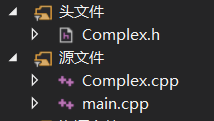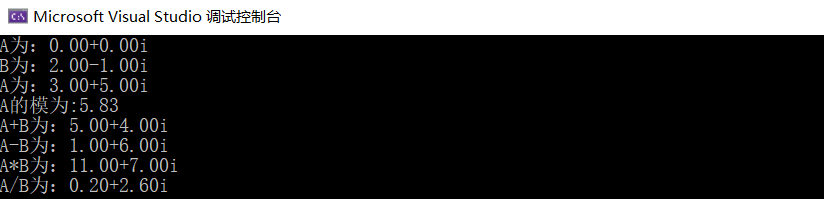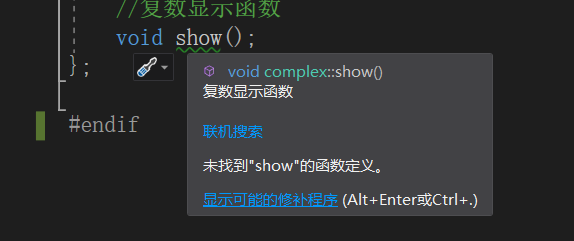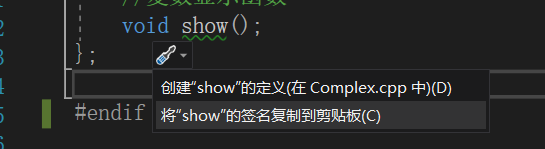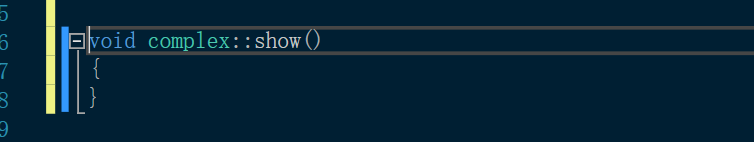09-09515304-042574
07-071491
05-116620
02-262489
03-114万+
03-271万+
09-141630
10-314万+
08-17528
05-042649
10-153415
02-141079
10-119352
08-284860点击重新获取扫码支付余额充值